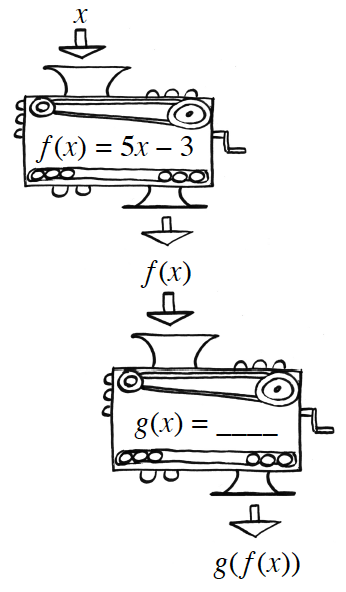### Home > PC3 > Chapter 1 > Lesson 1.2.1 > Problem1-74

1-74.Consider the function $f(x)=5x-3$

1. Describe, in words, what the function does to any input.

It multiplies the input by ___, then ___.

2. List steps, in order, that will “undo” the function.

This is the opposite steps in the reverse order from part (a).

3. Write an equation for the steps you wrote in part (b).
Name this equation $g$.

$g(x)=\frac{x+3}{5}$

4. What happens if you compose $f$ and $g$? Use the function machine diagram at right to evaluate $g(f(5))$. Explain why your answer makes sense.

What happens if you "do" something to a number and then "undo" it?